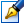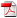##Lie algebras and algebras of invariants in study of differential systems

 Author: Elena Staruş Degree: doctor of physics and mathematics Speciality: 01.01.02 - Differential equations Year: 2005 Scientific adviser: Mihail Popadoctor habilitat, professor, Institute of Mathematics and Computer Science Institution: Institute of Mathematics and Computer Science Scientific council: DH 30-01.01.02-27.03.08 Moldova State University

### Status

The thesis was presented on the 3 December, 2004
Approved by NCAA on the 27 January, 2005

### Abstract1.24 Mb / in romanian

### Keywords

Lie algebra of operators, optimum system of subalgebras. invariants and comitants of differential systems, GL(2.R)-orbit, Hilbert series, dimension of Krull, minimal polynomial basis, factorsystem, non-singular invariant manifold of group GL(2.R), invariant GL(2.R)-integral.

### Summary

In the thesis is considered the system of type s(T) (T C {0,1. 2.3})

Arj

dx(j)/dt=a(j)+ а(j)ха + а(j)хах0 + а(j)хах?х, (j, а, b, y = 1, 2), (1)

where coefficient tensors a(j)ap and a3Qp are symmetrical in lower indexes in which the complete convolution takes place here.

The work is devoted to study of application of Lie algebras of operators and algebras of invariants to investigation of systems (1). The minimal polynomial basis of centro-affine comitants and invariants is built for systems s(0, 3) and s(0,1, 3), the classification of GL(2, R)-orbits' dimensions is done for systems s(0, 2) and s(0, 3). the characteristics of Lie algebra, admitted by system (1). are obtained. The generating functions and Hilbert series are built for algebras of unimodular comitants and invariants for system s(0, 3). and the dimension of Krull is found for these algebras.

In present work factorsystems are built for systems s(0,1), s(0, 1, 2) and s(0, 1, 2, 3), as well as first centro-affine invariant integrals for system s(0, 1) and particular centro-affine invariant integrals for system s(1, 2) on non-singular invariant manifolds of group GL(2, R).